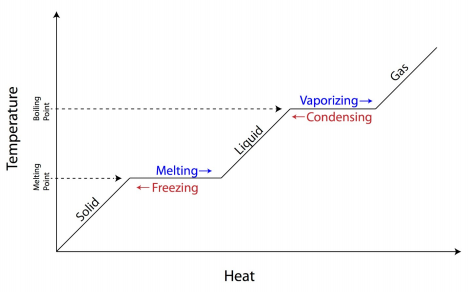×
Log in to StudySoup
Get Full Access to NMU - CHEM 111 - Study Guide - Midterm
Join StudySoup for FREE
Get Full Access to NMU - CHEM 111 - Study Guide - Midterm

Already have an account? Login here
×
Reset your password

NMU / Chemistry / CHEM 111 / 1.23 atm to mmhg

# 1.23 atm to mmhg Description

##### Uploaded: 10/25/2016
5 Pages 183 Views 0 Unlocks
Reviews

## What is the volume of the balloon at 20,000 m where atmospheric pressure is 63 mmHg and the temperature is 220K?## 0 L at a temperature of 273 K, what will the new volume be if the temperature is raised to 546 K?## 0 C, what will the final ᵒ ᵒ temperature of the water be?CH 111: Exam 3 Study Guide Highlight = Important Equation  Highlight = Important Concept  Highlight = Key Term Chapter 5: Thermochemistry Kinetic Energy ∙     (KE) the energy of movement   KE If you want to learn more check out asu mat 275
Don't forget about the age old question of randall osborne texas state university
Don't forget about the age old question of ego defensive function
If you want to learn more check out bronzing anatomy
If you want to learn more check out weber state criminal justice
We also discuss several other topics like lipids quiz
= ½ mv2 m = mass of molecules v = average velocity of molecules  KE is proportional to temperature (higher temperature = higher KE) Potential Energy ∙     aka. Electrostatic Potential Energy (Eel)  Eel = (Q1 x Q2)/dQ1 = charge of species 1 Q2 = charge of species 2 d = distance between species Chemical Systems ∙ Open System: can exchange matter and energy with its surroundings ∙ Closed System: can exchange energy but not matter with its surroundings ∙ Isolated System: cannot exchange matter or energy with its surroundings  Purely hypothetical, but we approximate it over short periods of time Internal Energy ∙ (E) the sum of KE and Eel  ∆E = Efinal – Einitial (change in internal energy)  *The sign (+ or ­) is important* ∙ ∆E = q + w q = heat change w = work = ­P∆V P = pressure ∆V = change in volume ∙ Joule: (J) 4.184 J = 1 cal Change in Enthalpy ∙  (∆H) refers to the amount of energy released or absorbed in a chemical reaction  At constant pressure (open system) q = qp qp = ∆H ∆H = qp = q + P∆V  Exothermic: gives off heat, forms stronger bonds in the products  ∆H = (­)  Endothermic: absorbs heat, forms stronger bonds in the reactants  ∆H = (+)  A gas forming reaction produces 12.0 L of a gas against a constant pressure of  1.05 atm. Calculate the work done by the gas in joules.  w = ­P∆V = ­(1.05 atm)(12.0L – 0L) = ­12.6 L*atm 101 J = 1 L*atm ­12.6 L*atm (101 J/1 L*atm) = ­1.27x103 JHeating Curve (Water) 100ᵒC ∆Hvaporization = 40.67kJ/mol ∆Hcondensation = - 40.67  ∆H kJ/mol fusion = 6.01  kJ/mol ∆Hsolidification = - 6.01  kJ/molHeat Capacity ∙ Molar heat capacity: (cp) (units: J/mol* C) amount of energy required to raise 1 mole of a  ᵒ substance by 1  Cᵒ  q = n*cp*∆T n = # of moles ∆T = Tfinal – Tinitial ( C) ᵒ ∙ Specific heat: (cs) (units: J/g* C) amount of energy required to raise 1 gram of a substance by  ᵒ 1 Cᵒ  q = m*cs*∆T m = mass (grams) ∙ Heat capacity: (Cp) (units: J/ C) amount of energy required to raise the temperature of a piece of ᵒ equipment by 1 Cᵒ  q = Cp*∆T ∙ Practice Problems  If 25.0 g Cu at 90.0 C is placed in 100.0 g of water at 20.0 C, what will the final  ᵒ ᵒ temperature of the water be? (cp(Cu) = 24.4 J/mol* C) ᵒ  Assume Tfinal is the same for both Cu and H2O  Assume container absorbs negligible heat   q → Cu = ­qH2O  25.0 g Cu (1 mol Cu/63.55g) = 0.3934 mol Cu 100.0 g H2O (1 mol H2O/18.02 g) = 5.5494 mol H2O  cp(Cu)*n*∆T = ­( cp(H2O)*n*∆T) (0.3934 mol)(24.4 J/mol* C)(T ᵒ f – 90.0 C) = ­(5.54 ᵒ 94 mol)(75.3 J/mol* C)(T ᵒ f – 20.0 C) ᵒ 9.599 J/ C(T ᵒ f – 90.0 C) = ­41 ᵒ 7.87 J/ C(T ᵒ f – 20.0 C) = ᵒ 9.599 J/ C(T ᵒ f) – 863.9 J = ­417.87 J/ C(T ᵒ f) – 8357 J = 427.5 J/ C(T ᵒ f) = 9221 J Tf = 21.6 Cᵒ  A 2.50 g sample of Zn at 22.5 C is added to 100.0 mL of a 2.0 M HCl solution at 22.5 C  ᵒ ᵒ in a calorimeter at 22.5 C with a total heat capacity of 481 J/ C. The observed  ᵒ ᵒ temperature of the system increases to 34.7 C. Calculate  ᵒ ∆HRxn for the following  equation in kJ/mol Zn: Zn(s) + 2HCl(aq)   ZnCl → 2(aq) +H2(g)  qRxn = ­qcalorimeter qRxn = ∆HRxn ∆HRxn = ­qcalorimeter  ∆HRxn = ­(481 J/ C)(34.7 C – 22.5 C) = ­58 ᵒ ᵒ ᵒ 68 J = ­5.868 kJ  2.50 g Zn (1 mol Zn/65.39 g) = 0.03823 mol Zn ←limiting reactant 2.0 mol/L HCl (0.1000 L) = 0.20 mol HCl/2 = 0.10 mol Zn  ­5.868 kJ/0.03823 mol Zn = ­153 kJ/ mol Zn Standard Enthalpy of Formation ∙  (∆Hᵒf) the heat change associated with the formation of a substance from the elements (*Values  will be given to you for the test*)  ∆Hᵒf  CO2(g) = C(s) + O2(g)   CO → 2(g) ∆HᵒRxn = ∆Hᵒf  (∆Hᵒf for elements is 0)  ∆HᵒRxn = Sum ∆Hᵒf (Products) – Sum ∆Hᵒf (Reactants) ∙ Calculate ∆HᵒRxn for 2C2H2(g) + 5O2(g)   4CO → 2(g) + 2H2O(g) ∆Hᵒf (kJ/mol):        226.7 0 ­393.5     ­241.8  [4(­393.5) + 2(­241.8)] – [2(226.7) + 5(0)] = ­2511 kJ/mol (Products)             ­  (Reactants) Hess’s Law ∙ If 2 or more chemical equations are added together, then the value of ∆HRxn (or ∆HᵒRxn) for the  resulting equation is the sum of the ∆HRxn (or ∆HᵒRxn) values for the separate equations ∙ ClO(g) + O3(g)   Cl → (g) + 2O2(g) ∆HᵒRxn = ­29.90 kJ/mol 2O3(g)   3O → 2(g) ∆HᵒRxn = 24.18 kJ/mol Calculate ∆HᵒRxn for:  Cl(g) + O3(g)   ClO → (g) + O2(g)  Flip [ClO(g) + O3(g)   Cl → (g) + 2O2(g)] so the O3 and O2 cancel out correctly with the other  equation       *When you flip an equation, change the sign for the ∆HᵒRxn value*     Cl(g) + 2O2(g)   ClO → (g) + O3(g)    (+)29.90  +           2O3(g)   3O → 2(g) +     24.18  Cl(g) + O3(g)   ClO → (g) + O2(g) 54.08 kJ/mol Chapter 6: Properties of Gases Ideal Gas Law ∙     PV = nRT (*memorize*)  P = pressure (usually atm)   V = volume (L)  n = moles of gas (mol)  R = gas constant (usually atm*L/mol*K)  R = 0.08206 atm*L/mol*K  (*You don’t need to memorize this constant*) T = temperature (K) ∙ Conditions for an Ideal Gas  The volume of gas particles is minimal relative to the volume of the gas sample  Gas particles have no attraction to each other  *We will always assume gases are ideal in this unit* Laws Derived from Ideal Gas Law ∙ P1V1 = P2V2 (units don’t matter as long as they are the same on both sides)  There is a gas sample with a volume of 2.0 L and a pressure of 4.0 atm, what will  the new volume be if the pressure is increased to 8.0 atm? (2.0 L)(4.0 atm) = V2(8.0 atm) V2 = 1.0 L ∙ V1/T1 = V2/T2 (units don’t matter as long as they are the same on both sides)  There is a gas sample with a volume of 2.0 L at a temperature of 273 K, what will  the new volume be if the temperature is raised to 546 K? (2.0 L)/(273 K) = V2/(546 K) V2 = 4.0 L Practice Problems ∙ A weather balloon with a volume of 250.0 L is launched at 21oC at sea level, where the  atmospheric pressure is 1.00 atm. What is the volume of the balloon at 20,000 m where  atmospheric pressure is 63 mmHg and the temperature is 220K?  PV = nRT P1V1/T1 = P2V2/T2 V2 = P1V1T2/T1P2 V1 = 250.0 L V2 = ? T1 = 21oC + 273.15 = 294.15 K T2 = 220 K P1 = 1.00 atm P2=63 mmHg(1 atm/760 mmHg) = 8.29x10­2 atm  V2 = (1.00 atm)(250.0 L)(220K)/(294.15 K)(8.29x10­2 atm) = 2.26 x 103 L ∙ Acid precipitation dripping on limestone produces carbon dioxide by the following  equation: CaCO3(s) + 2 H+(aq)   Ca → 2+(aq) + CO2(g) + H2O(l) If 25.0 mL of CO2 were produced at  25oC and 752 mmHg then how many milligrams of CaCO3 were consumed?  n = PV/RT V = 0.0250 L P = 756 mmHg (1 atm/760 mmHg) = 0.9895 atm T = 25oC + 273= 298 K  n =(0.9895 atm)(0.0250 L)/(0.08206 atm*L/mol*K)(298 K) = 1.012 x 10­3 mol CO2  1.012 x 10­3 mol (1 mol CaCO3/1 mol CO2)(100.09 g/1 mol CaCO3)(1000 mg/1 g) = 101  mg CaCO3 Dalton’s Law of Partial Pressures ∙ Total pressure is equal to the sum of the partial pressures of the component gases  PTotal = P1 + P2 + P3 … +Pn ∙ A 2.0 L sample of H2(g) at 1.00 atm, an 8.0 L sample of N2(g) at 3.00 atm, and 4.0 L sample of  Kr(g) at 0.50 atm are transferred to a single 10.0 L container at the same temperature.  Calculate the partial pressure of each gas and the total final pressure in the new container.  P1V1 = P2V2 PT = PH2 + PN2 + PKr H2: P1 = 1.00 atm      V1 = 2.0 L       V2 = 10.0 L  P2 = 0.20 atm N2: P1 = 3.00 atm      V1 = 8.0 L       V2 = 10.0 L  P2 = 2.4 atm Kr: P1 = 0.50 atm      V1 = 4.0 L       V2 = 10.0 L  P2 = 0.20 atm  PT = 0.20 atm + 2.4 atm + 0.20 atm = 2.8 atm ∙ Lithium metal reacts with N2(g) at room temperature (20oC) according to the equation:   6 Li(s) + N2(g)   2 Li → 3N(s) A sample of Li(s) was placed under a N2(g) atmosphere in a sealed 1.00 L container at a  pressure of 1.23 atm. One hour later the pressure had dropped to 0.92 atm. Calculate the number of grams of N2(g) that reacted with the Li(s). Assuming that all the Li(s) reacted,  calculate the mass of Li(s) originally present.  1.23 atm – 0.92 atm = 0.31 atm N2 reacted  n = PV/RT  (0.31 atm)(1.00 L)/( 0.08206 atm*L/mol*K)(293K) = 1.29 x 10­2 mol N2 1.29 x 10­2 mol N2 (28.02 g/1 mol N2) = 0.36 g N2  1.29 x 10­2 mol N2 (6 mol Li/1 mol N2)(6.94 g/1 mol Li) = 0.54 g Li Mole Fraction ∙ (X) concentration units used for gas mixtures  X = # of moles of component/total # of moles *memorize*  Pn = PTotal * Xn *memorize* ∙ A 0.622 g mixture of gases contained in vessel at 1450 mmHg is found to be 25.0% argon  and 75.0% chlorine by mass. What is the partial pressure of each of the gases in the  mixture?  PT = PCl2 + PAr PAr = XAr * PT XAr = mol Ar/(mol Ar + mol Cl2) PCl2 = XCl2 * PT XCl2 = mol Cl2/(mol Ar + mol Cl2)  Ar: 0.250(0.622 g) = 0.1555 g Ar (1 mol Ar/39.95 g) = 3.892 x 10­3 mol Ar Cl2: 0.622 g – 0.1555 g = 0.4665 g Cl2 (1 mol Cl2/70.90 g) = 6.5797 x 10­3 mol Cl2  XAr = 3.892 x 10­3 mol Ar/(3.892 x 10­3 mol + 6.5797 x 10­3 mol) = 0.3717 XCl2 = 6.5797 x 10­3 mol Cl2/(3.892 x 10­3 mol + 6.5797 x 10­3 mol) = 0.6283  PAr = 0.3717(1450 mmHg) = 539 mmHg PCl2 = 0.6283(1450 mmHg) = 911 mmHg Kinetic Molecular Theory 1. As temperature increases, average speed of gas particles increases 2. At a given temperature, lighter gas molecules are moving faster than heavier gas molecules ∙ At higher temperatures, the range of speeds is greater

Page ExpiredIt looks like your free minutes have expired! Lucky for you we have all the content you need, just sign up here
References: